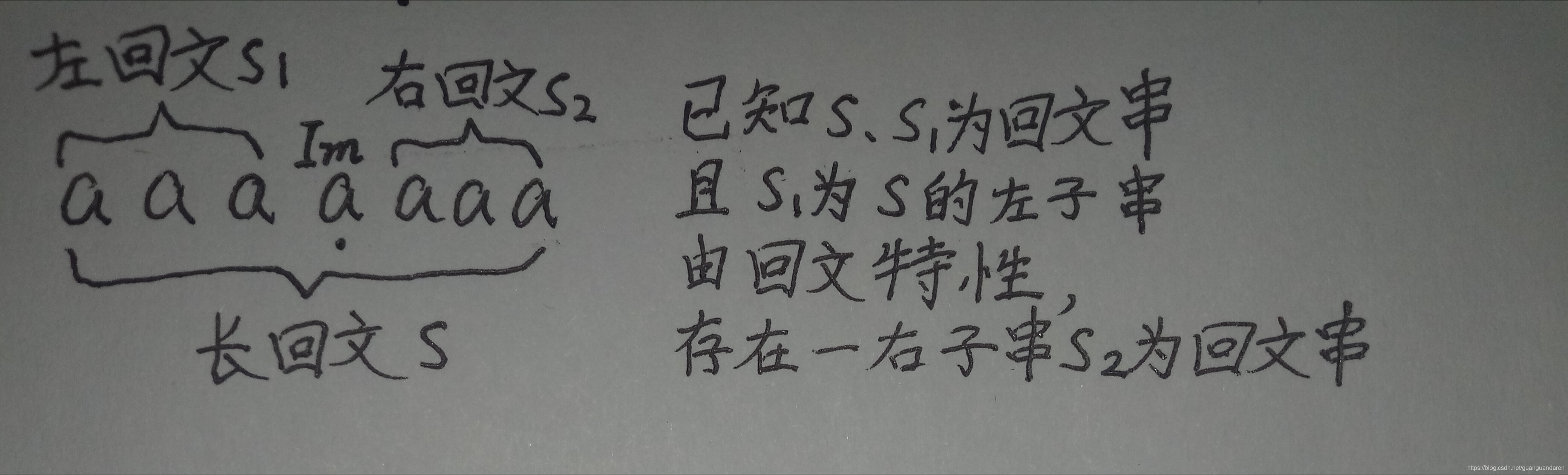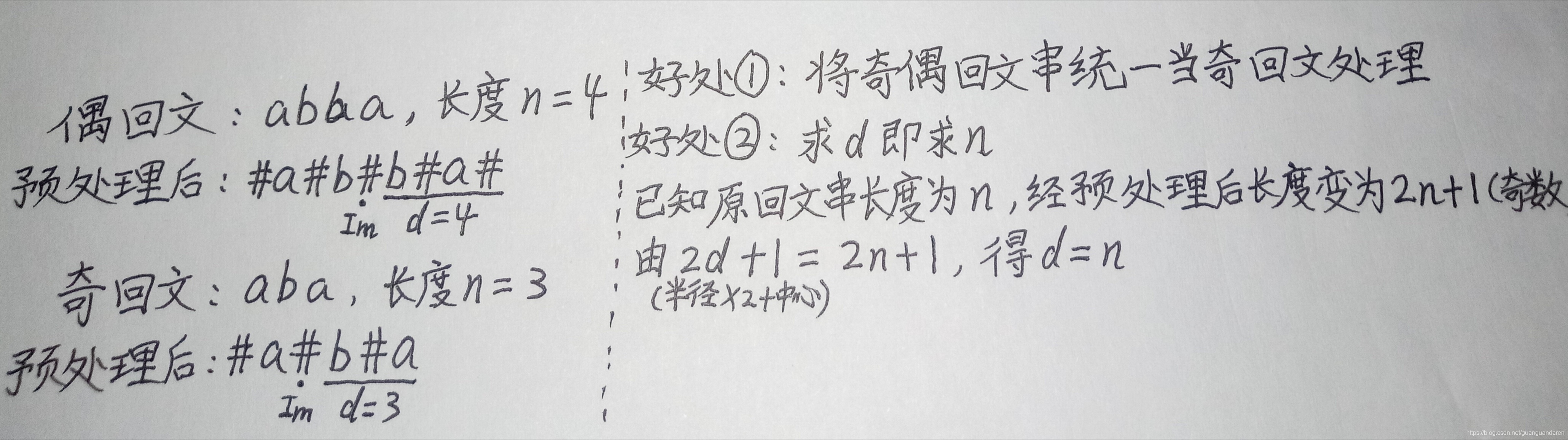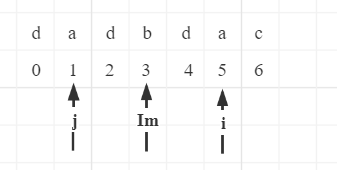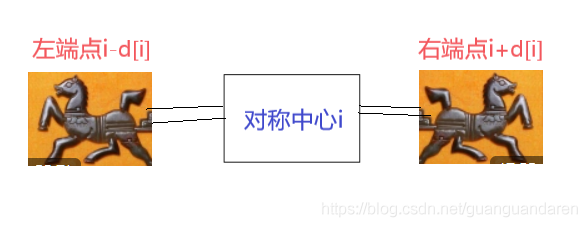Kubrnete309 0 0

## 中心拓展法

//遍历2n-1次，就能够得到所有可能的对称中心
for (int i = 0; i < 2 * n - 1; i++) {
int l = i / 2, r = i / 2 + i % 2;//这样能够把长度为奇数和偶数的两种情况统一起来。
while (l >= 0 && r < n && s[l] == s[r]) {
--l;
++r;
++ans;
}
}### 1. 长回文串中的重复查找(关键)：## 马拉车算法：

### 二、算法实现：

1.预处理(解决了奇偶回文串问题)：

• 为了利用回文串的对称性，同时解决回文子串长度有奇数和偶数两种情况的问题，马拉车算法在字符串首尾及每个字符间都插入一个 "#"。可以发现，无论回文子串原来是奇数还是偶数，最终都会变成奇回文子串，因此能够统一处理。即令原字符串为s,预处理后有 ts = s.replace('', '#')

• 我们定义①

当前向两边拓展距离最大的回文串为最大回文串，即一个长回文串；②

从对称中心到左端点的距离为最大回文半径，并假设为d；

当我们求出最大回文半径d时，

最大回文串

的长度正好是d。• 另外，在下面的步骤中，为了避免数组越界，我们还要在字符串开头加一个"$"，结尾加一个"!"，这样开头和结尾的两个字符一定不相等，循环就可以在这里终止。 预处理代码如下 s = '$' + input().replace('', '#') + '!'
#为了避免数组越界，让开头和结尾的两个字符一定不相等，循环就可以在这里终止。2.初始化(解决了重复查找问题):

• 数据结构与逻辑变量:马拉车算法的要求维护的变量有，一个数组d[]记录最大回文半径,d[i]表示以第i位元素为对称中心的最大回文半径，初始化长度为0(半径算上中心就是1)；当前最大回文串的(即长回文串)对称中心Im及其右端点r，初始化皆为0。
• 初始化
• 以字符串"dadbdac"为例• Case 1: i<=r，即当前枚举的对称中心i被包含在当前最大回文串内，此时拓展过程可以加速
1. 当找到一个长回文串时，取其对称中心为Im，对于其中的每个对称中心i，我们可以求出关于Im的对称位置 j=2*Im-i
2. 在这个长回文串范围内，可以通过对称性得到d[i]=d[j] 。
3. 注意当d[j]>r-i时，通过d加速拓展的右子串超出了长回文串的范围。然而大于右端点的回文串我们还没有开始匹配，此时d[i] = r - i . 比方说，当Im=3时,有r=5;当i=5时,有j=1,并且前面已经算出d[j] = 1。此时d[j]>r-i，向右拓展超出了长回文串范围，则d[i]应为i到右端点r的距离,即r-i。因此，当对称中心i被包含在当前最大回文串内时，有

d[i]=min(d[2 * Im - i],r - i) （核心：如果d[j]+i<=r,则d[i]=d[j],否则d[i]=r-i）

• Case 2 : i>r\，此时用常规的中心拓展法更新d[]、Im以及r。

3.中心拓展

for i in range(2, len(s)-2):
if(i<=r):#当对称中心i被包含在当前最大回文串内时，拓展过程可以加速(核心)
d[i]=min(d[2 * Im - i] , r - i )
while (s[i - d[i] - 1] == s[i + d[i] + 1]):#满足条件，向左右拓展半径
d[i] += 14.维护当前最大回文串的对称中心Im和r

s $# d # a # d # b # d # a # c # ! i 0 1 2 3 4 5 6 7 8 9 10 11 12 13 14 15 16 d[i] 1 0 3 0 1 0 5 0 1 0 1 0 1 其实在上述的核心思路中，在枚举每个回文子串的对称中心时，也在不断匹配最大回文串并更新Im和r。利用已经算出来的状态来更新d[i]，这就是一种动态规划的思想 对于一个回文串，我们用d[i]记录了其对称中心到右端点的距离。以上面的例子来说明，当i=8时(属于i>r的情况)，计算出d[i]=5，则此时容易得到r=i+d[i]=13,同时更新Im=8。对于i<=r的情况，如果i+d[i]>r，说明当前对称中心i拓展出了回文串，于是此时需要更新对称中心Im为i,右端点r为i+d[i]。而对于i>r的情况,由于d[i]总是等于0，此时更新Im=i,r=i if (i + d[i] > r): Im, r = i, i + d[i]顺带提一句，最开始我以为马拉车这个算法名字应该是根据Manacher英译而来，但是这个中心扩展的思路，加上通过d[i]记录了最大回文串的半径避免了重复匹配，光看这两行代码： while (s[i - d[i] - 1] == s[i + d[i] + 1]) d[i]++; if (i + d[i] > r){Im=i, r = i + d[i];}真是有两头马在拉着一个对称中心向两边跑的感觉，大概就像这样：)然后不断更新最大回文串的对称中心Im和 r，通过***d[i] = min(d[2 \ Im - i], r - i)快速求解** 如何求解ans就不解释啦，直接上代码: s = '$' + input().replace('', '#') + '!'
d,Im,r,ans= *(len(s)-2),0,0,0
for i in range(1, len(s)-2):
if (i <= r):#当对称中心i被包含在当前最大回文串内时，拓展过程可以加速
d[i] = min(d[2 * Im - i], r - i) #核心：如果d[j]+i<=r,则d[i]=d[j],否则d[i]=r-i
while (s[i - d[i] - 1] == s[i + d[i] + 1]):#满足条件，向左右拓展半径
d[i] += 1
if (i + d[i] > r):
Im, r = i, i + d[i]
ans += (d[i] + 1) // 2
print(ans)ma,ans = (d[i],ts[i-d[i]:i+d[i]]) if (d[i]>ma) else (ma,ans)
print(ans.replace('#',''))Python正则表达式 2021-03-13 17:43